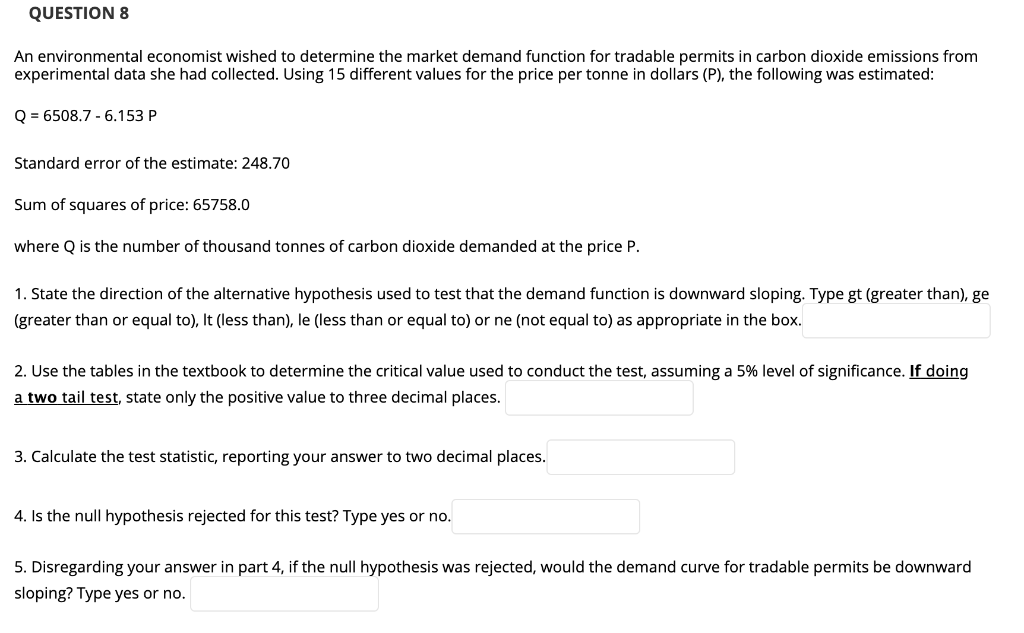# Question QUESTION 8 An environmental economist wished to determine the market demand function for tradable permits in carbon dioxide emissions from experimental data she had collected. Using 15 different values for the price per tonne in dollars (P), the following was estimated: Q = 6508.7 - 6.153 P Standard error of the estimate: 248.70 Sum of squares of price: 65758.0 where Q is the number of thousand tonnes of carbon dioxide demanded at the price P. 1. State the direction of the alternative hypothesis used to test that the demand function is downward sloping. Type gt (greater than), ge (greater than or equal to), It (less than), le (less than or equal to) or ne (not equal to) as appropriate in the box. 2. Use the tables in the textbook to determine the critical value used to conduct the test, assuming a 5% level of significance. If doing a two tail test, state only the positive value to three decimal places. 3. Calculate the test statistic, reporting your answer to two decimal places. 4. Is the null hypothesis rejected for this test? Type yes or no. 5. Disregarding your answer in part 4, if the null hypothesis was rejected, would the demand curve for tradable permits be downward sloping? Type yes or no.MIEWCJ The Asker · Probability and StatisticsTranscribed Image Text: QUESTION 8 An environmental economist wished to determine the market demand function for tradable permits in carbon dioxide emissions from experimental data she had collected. Using 15 different values for the price per tonne in dollars (P), the following was estimated: Q = 6508.7 - 6.153 P Standard error of the estimate: 248.70 Sum of squares of price: 65758.0 where Q is the number of thousand tonnes of carbon dioxide demanded at the price P. 1. State the direction of the alternative hypothesis used to test that the demand function is downward sloping. Type gt (greater than), ge (greater than or equal to), It (less than), le (less than or equal to) or ne (not equal to) as appropriate in the box. 2. Use the tables in the textbook to determine the critical value used to conduct the test, assuming a 5% level of significance. If doing a two tail test, state only the positive value to three decimal places. 3. Calculate the test statistic, reporting your answer to two decimal places. 4. Is the null hypothesis rejected for this test? Type yes or no. 5. Disregarding your answer in part 4, if the null hypothesis was rejected, would the demand curve for tradable permits be downward sloping? Type yes or no.
More
Transcribed Image Text: QUESTION 8 An environmental economist wished to determine the market demand function for tradable permits in carbon dioxide emissions from experimental data she had collected. Using 15 different values for the price per tonne in dollars (P), the following was estimated: Q = 6508.7 - 6.153 P Standard error of the estimate: 248.70 Sum of squares of price: 65758.0 where Q is the number of thousand tonnes of carbon dioxide demanded at the price P. 1. State the direction of the alternative hypothesis used to test that the demand function is downward sloping. Type gt (greater than), ge (greater than or equal to), It (less than), le (less than or equal to) or ne (not equal to) as appropriate in the box. 2. Use the tables in the textbook to determine the critical value used to conduct the test, assuming a 5% level of significance. If doing a two tail test, state only the positive value to three decimal places. 3. Calculate the test statistic, reporting your answer to two decimal places. 4. Is the null hypothesis rejected for this test? Type yes or no. 5. Disregarding your answer in part 4, if the null hypothesis was rejected, would the demand curve for tradable permits be downward sloping? Type yes or no.